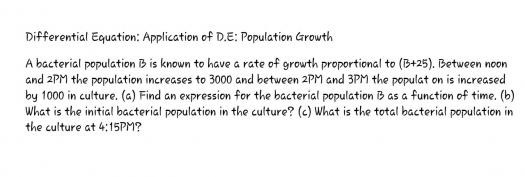# Differential Equation: Application of D.E: Population Growth### Differential Equation:

Differential Equation: Application of D.E.: Population Growth
A bacterial population B is known to have a rate of growth proportional to (B + 25). Between noon and 2PM the population increases to 3000 and between 2PM and 3PM the population is increased by 1000 in culture. (a) Find an expression for the bacterial population B as a function of time. (b) What is the initial bacterial population in the culture? (c) What is the total bacterial population in the culture at 4:15PM?### $\dfrac{dB}{dt} = k(B + 25)$

$\dfrac{dB}{dt} = k(B + 25)$

$\dfrac{dB}{B + 25} = k \, dt$

$\displaystyle \int \dfrac{dB}{B + 25} = k \int dt$

$\ln (B + 25) = kt + C$

At 2:00PM, t = 2 and B = 3000
$\ln 3025 = 2k + C$   ←   eq. (1)

At 3:00PM, t = 3 and B = 4000
$\ln 4025 = 3k + C$   ←   eq. (2)

From eq. (1) and eq. (2)
$k = 0.2856$

$C = 7.4434$

Hence,
$\ln (B + 25) = 0.2856t + 7.4434$       answer for (a)

At noon, t = 0
$\ln (B + 25) = 7.4434$

$B = 1683$       answer for (b)

At 4:15PM, t = 4.25
$\ln (B + 25) = 0.2856(4.25) + 7.4434$

$B = 5726$       answer for (c)### Another solution (By

Another solution (By Calculator - CASIO fx-991ES PLUS):
MODE 3 5

X Y
2 3000 + 25
3 4000 + 25

AC
$B + 25 = 0\hat{y}$

$B + 25 = 1708$

$B = 1683$       answer for (b)

$B + 25 = 4.25\hat{y}$

$B + 25 = 5751$

$B = 5726$       answer for (c)

Note:
$\hat{y}$ = SHIFT 1 5 5

• Mathematics inside the configured delimiters is rendered by MathJax. The default math delimiters are $$...$$ and $...$ for displayed mathematics, and $...$ and $...$ for in-line mathematics.